## Overview¶

Networkx is a python library for creation, manipulation and understanding structure of complex networks. It supports the creation of graphs, digraphs & multigraphs. It also has various graph algorithms by default.

Networkx supports nodes like text, images, XML and edges like time-series, weight, etc.

It can be used to analyze the structure of social, biological, infrastructure and various other types of networks.

In :
import numpy as np # linear algebra
import pandas as pd # data processing, CSV file I/O (e.g. pd.read_csv)
import matplotlib.pyplot as plt
import os
import sys
import networkx as nx
import scipy

print('Python Version : '+sys.version)
print('NetworkX version : '+nx.__version__)

Python Version : 3.6.5 |Anaconda, Inc.| (default, Apr 29 2018, 16:14:56)
[GCC 7.2.0]
NetworkX version : 2.2


Users can test networkx library by running its tests using the below statements. I have commented on it as of now as It'll take time to run.

In :
#nx.test()


Undirected Graphs can be created using simple Graph constructor. The graph consists of a list of nodes and node pairs (edges). Any hashable object except None can be node of graph-like text, image, etc. including another graph as well.

It allows self-loop for node but it does not allow parallel edges (more than 1 edges between 2 nodes).

Graph constructor can be initialized in various ways mentioned below:

• Empty without any node
• Using list of edges
• Using dict of dict
• dict of lists
• Using Numpy matrix / 2-d array
• Using scipy sparse array
• Other networkx graph
In :
G = nx.Graph()
G1 = nx.Graph([(4,5),(6,7),(8,9), (5,7),(4,9), (9,7)]) ## Creating graphs from list of edges
G2 = nx.Graph(G1) ## Creating graph from existing graph
G3 = nx.Graph(dict([(4,[(6,7),(2,3)]),(6,(8,9)), (5,(5,7)),(7,(4,9)), (9,(9,7))])) ## Creating graph from dict of list
G4 = nx.Graph(dict([(4,{6:7}),(6,{10:11}), (5,{5: 7}),(7,{4: 9}), (9,{9:7})])) ## Creating graph from dict of dict
l = np.random.rand(5,5)
print(l)
G5 = nx.Graph(l) ## Creating graph from numpy 2-d array
row = [0,3,1,0]
col = [0,3,1,2]
data = [4,5,7,9]
sparse_mat = scipy.sparse.coo_matrix((data, (row, col)), shape=(4,4)) ## Creating graph from scipy sparse matrix
print(sparse_mat.toarray())
G6 = nx.Graph(sparse_mat)

plt.figure(figsize=(12,3))
plt.subplot(1,6,1)
nx.draw(G1, with_labels=True, font_weight='bold')
plt.title('G1')
plt.subplot(1,6,2)
nx.draw(G2, with_labels=True, font_weight='bold')
plt.title('G2')
plt.subplot(1,6,3)
nx.draw(G3, with_labels=True, font_weight='bold')
plt.title('G3')
plt.subplot(1,6,4)
nx.draw(G4, with_labels=True, font_weight='bold')
plt.title('G4')
plt.subplot(1,6,5)
nx.draw(G5, with_labels=True, font_weight='bold')
plt.title('G5')
plt.subplot(1,6,6)
nx.draw(G6, with_labels=True, font_weight='bold')
plt.title('G6')
None

[[0.60065143 0.55016422 0.84584434 0.05650226 0.54877556]
[0.48530079 0.82989731 0.06787776 0.30957781 0.13379917]
[0.83520381 0.74233977 0.49858038 0.72948412 0.32426165]
[0.31484124 0.43817928 0.89526937 0.29585088 0.36491087]
[0.88816161 0.84510195 0.71088983 0.04815844 0.41441356]]
[[4 0 9 0]
[0 7 0 0]
[0 0 0 0]
[0 0 0 5]]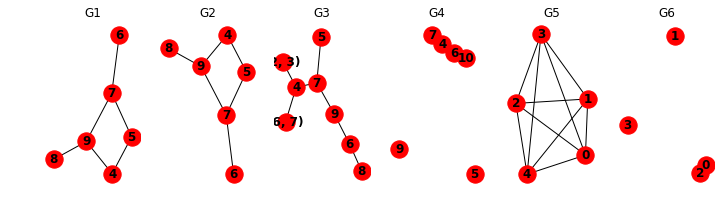Below we are analyzing properties of graphs created above using various methods.

In :
for i, graph in enumerate([G1,G2,G3,G4,G5,G6], start=1):
print('Graph%d : '%i)
print('Nodes : %s'%graph.nodes,'Edges : %s'%graph.edges)
print('Attribute with first edge : %s'%graph.get_edge_data(*list(graph.edges)))
print('Nodes with selfloops : %s'%list(graph.nodes_with_selfloops()))
print('Undirected : %s'%graph.is_directed())
print('Number of Edges : %s'%graph.number_of_edges())
print('Number of Nodes : %s'%graph.number_of_nodes())
print('Graph size : %s'%graph.size())
print('Degree of each node : %s'%graph.degree)
print(graph.name)

Graph1 :
Nodes : [4, 5, 6, 7, 8, 9] Edges : [(4, 5), (4, 9), (5, 7), (6, 7), (7, 9), (8, 9)]
Attribute with first edge : {}
Nodes with selfloops : []
Undirected : False
Number of Edges : 6
Number of Nodes : 6
Graph size : 6
Graph adjacent nodes : {4: {5: {}, 9: {}}, 5: {4: {}, 7: {}}, 6: {7: {}}, 7: {6: {}, 5: {}, 9: {}}, 8: {9: {}}, 9: {8: {}, 4: {}, 7: {}}}
Degree of each node : [(4, 2), (5, 2), (6, 1), (7, 3), (8, 1), (9, 3)]

Graph2 :
Nodes : [4, 5, 6, 7, 8, 9] Edges : [(4, 5), (4, 9), (5, 7), (6, 7), (7, 9), (8, 9)]
Attribute with first edge : {}
Nodes with selfloops : []
Undirected : False
Number of Edges : 6
Number of Nodes : 6
Graph size : 6
Graph adjacent nodes : {4: {5: {}, 9: {}}, 5: {4: {}, 7: {}}, 6: {7: {}}, 7: {5: {}, 6: {}, 9: {}}, 8: {9: {}}, 9: {4: {}, 7: {}, 8: {}}}
Degree of each node : [(4, 2), (5, 2), (6, 1), (7, 3), (8, 1), (9, 3)]

Graph3 :
Nodes : [4, 6, 5, 7, 9, (6, 7), (2, 3), 8] Edges : [(4, (6, 7)), (4, (2, 3)), (4, 7), (6, 8), (6, 9), (5, 5), (5, 7), (7, 9), (9, 9)]
Attribute with first edge : {}
Nodes with selfloops : [5, 9]
Undirected : False
Number of Edges : 9
Number of Nodes : 8
Graph size : 9
Graph adjacent nodes : {4: {(6, 7): {}, (2, 3): {}, 7: {}}, 6: {8: {}, 9: {}}, 5: {5: {}, 7: {}}, 7: {5: {}, 4: {}, 9: {}}, 9: {6: {}, 7: {}, 9: {}}, (6, 7): {4: {}}, (2, 3): {4: {}}, 8: {6: {}}}
Degree of each node : [(4, 3), (6, 2), (5, 3), (7, 3), (9, 4), ((6, 7), 1), ((2, 3), 1), (8, 1)]

Graph4 :
Nodes : [4, 6, 5, 7, 9, 10] Edges : [(4, 6), (4, 7), (6, 10), (5, 5), (9, 9)]
Attribute with first edge : {}
Nodes with selfloops : [5, 9]
Undirected : False
Number of Edges : 5
Number of Nodes : 6
Graph size : 5
Graph adjacent nodes : {4: {6: {}, 7: {}}, 6: {4: {}, 10: {}}, 5: {5: {}}, 7: {4: {}}, 9: {9: {}}, 10: {6: {}}}
Degree of each node : [(4, 2), (6, 2), (5, 2), (7, 1), (9, 2), (10, 1)]

Graph5 :
Nodes : [0, 1, 2, 3, 4] Edges : [(0, 0), (0, 1), (0, 2), (0, 3), (0, 4), (1, 1), (1, 2), (1, 3), (1, 4), (2, 2), (2, 3), (2, 4), (3, 3), (3, 4), (4, 4)]
Attribute with first edge : {'weight': 0.6006514325843043}
Nodes with selfloops : [0, 1, 2, 3, 4]
Undirected : False
Number of Edges : 15
Number of Nodes : 5
Graph size : 15
Graph adjacent nodes : {0: {0: {'weight': 0.6006514325843043}, 1: {'weight': 0.4853007875598152}, 2: {'weight': 0.8352038100134676}, 3: {'weight': 0.31484124366265387}, 4: {'weight': 0.8881616050771075}}, 1: {0: {'weight': 0.4853007875598152}, 1: {'weight': 0.8298973124186575}, 2: {'weight': 0.7423397742630208}, 3: {'weight': 0.4381792773910934}, 4: {'weight': 0.8451019482473585}}, 2: {0: {'weight': 0.8352038100134676}, 1: {'weight': 0.7423397742630208}, 2: {'weight': 0.4985803814665891}, 3: {'weight': 0.8952693726486124}, 4: {'weight': 0.710889830303774}}, 3: {0: {'weight': 0.31484124366265387}, 1: {'weight': 0.4381792773910934}, 2: {'weight': 0.8952693726486124}, 3: {'weight': 0.2958508832706138}, 4: {'weight': 0.04815844448820494}}, 4: {0: {'weight': 0.8881616050771075}, 1: {'weight': 0.8451019482473585}, 2: {'weight': 0.710889830303774}, 3: {'weight': 0.04815844448820494}, 4: {'weight': 0.4144135595202092}}}
Degree of each node : [(0, 6), (1, 6), (2, 6), (3, 6), (4, 6)]

Graph6 :
Nodes : [0, 1, 2, 3] Edges : [(0, 0), (0, 2), (1, 1), (3, 3)]
Attribute with first edge : {'weight': 4}
Nodes with selfloops : [0, 1, 3]
Undirected : False
Number of Edges : 4
Number of Nodes : 4
Graph size : 4
Graph adjacent nodes : {0: {0: {'weight': 4}, 2: {'weight': 9}}, 1: {1: {'weight': 7}}, 2: {0: {'weight': 9}}, 3: {3: {'weight': 5}}}
Degree of each node : [(0, 3), (1, 2), (2, 1), (3, 2)]



There are various methods available to add new nodes and edges to the graph. We have listed below a few of them. One can add any hashable python object as a node to the graph. One can add another graph as well as a node to an existing graph.

In :
plt.figure(figsize=(8,4))
G = nx.Graph()
print(G.get_edge_data(26,27), G.get_edge_data(28,29))
nx.draw(G, with_labels=True, font_weight='bold')

{'weight': 3.14} {'weight': 6.45}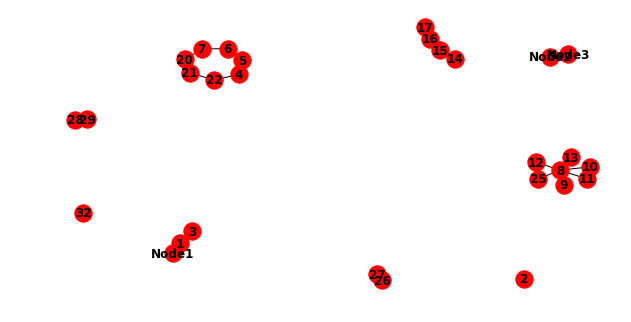In :
H = nx.path_graph(10)
G.remove_node(32), G.remove_node('Node2'), G.remove_node(28) ## Removing node 'Node2' & 28 also removed edges associated with them
G.remove_edge(26,27) ## Though edge 26-27 is removed but node 26,27 will still be existing independent
nx.draw(G, with_labels=True, font_weight='bold')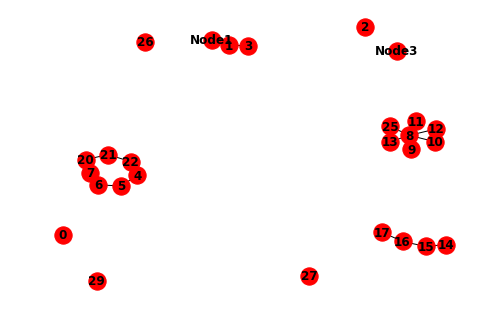In :
nx.draw_shell(G, with_labels=True, font_weight='bold')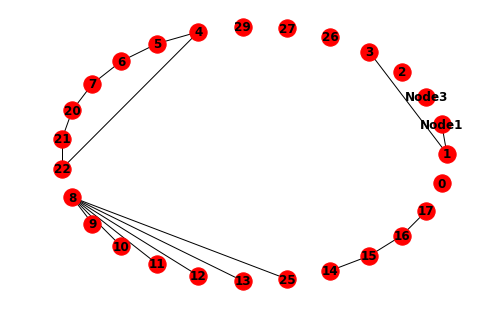In :
nx.draw_random(G, with_labels=True, font_weight='bold')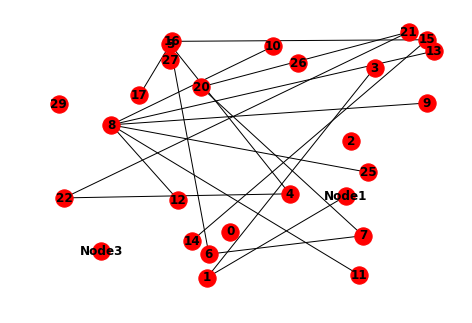In :
G = nx.dfs_tree(G)
nx.draw(G, with_labels=True, font_weight='bold')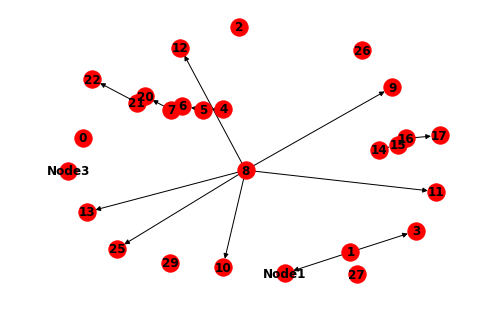In :
G = nx.bfs_tree(G,source=8)
nx.draw(G, with_labels=True, font_weight='bold')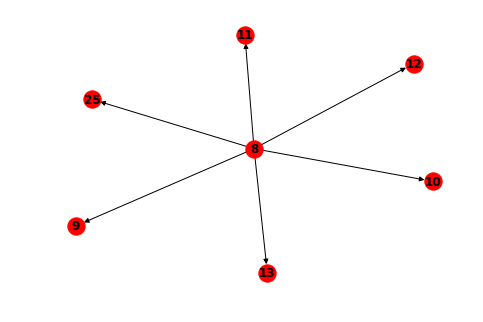In :
G = nx.dodecahedral_graph()
nx.draw(G, with_labels=True, font_weight='bold')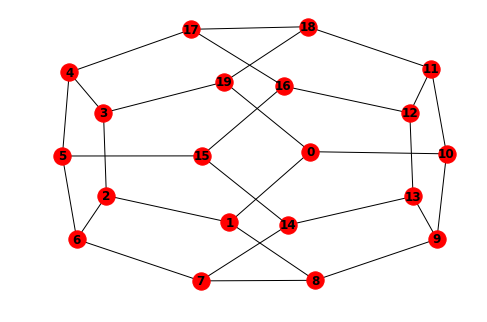In :
Di_G = nx.DiGraph(G)
nx.draw(Di_G, with_labels=True, font_weight='bold')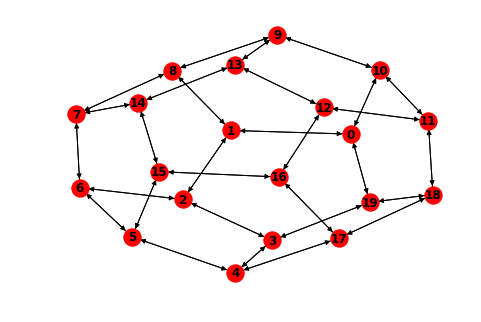In :
Di_G1 = nx.DiGraph(G1)
nx.draw(Di_G1, with_labels=True, font_weight='bold')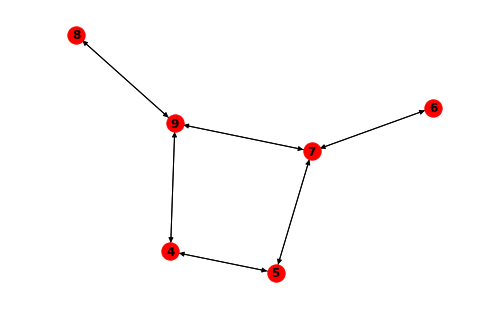Sunny Solanki